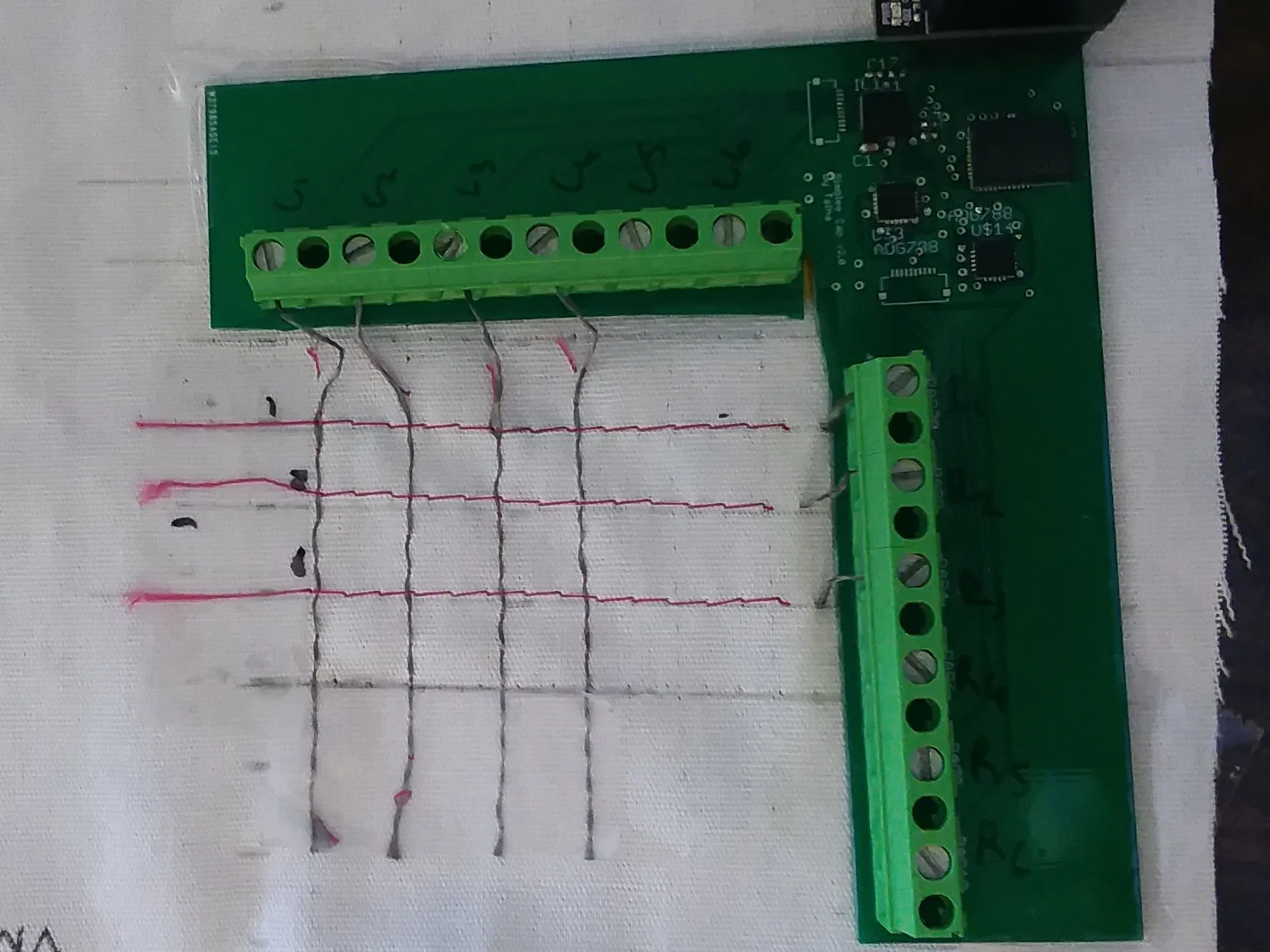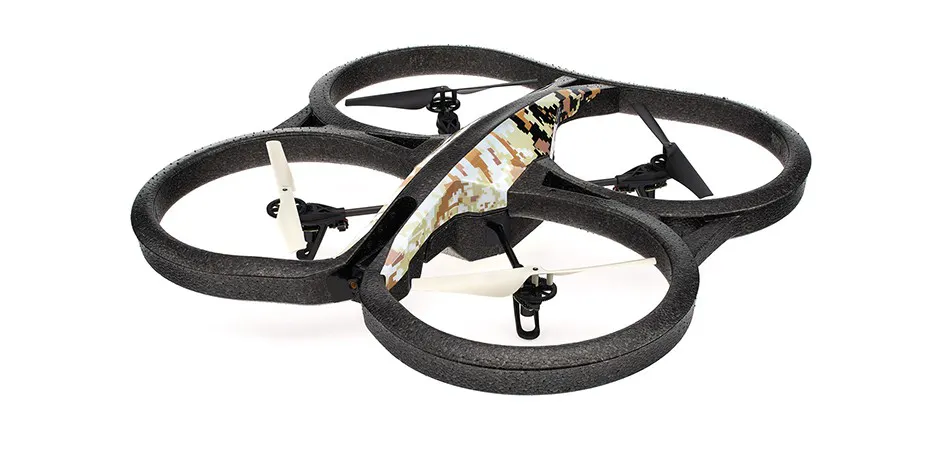# Textile-Based Capacitive Sensor Control of Parrot AR Drone

Interface a capacitive sensor with a computer, then use it to control a Parrot AR drone.

IntermediateFull instructions provided4 hours284## Things used in this project

### Hardware components

 textile based capacitive sensor
×1Parrot AR.Drone
×1

## Code

### Capacitive sensor subscriber

Python
paste this into any python file you would like
```import serial
import os
from geometry_msgs.msg import Twist
import rospy
import numpy as np
import matplotlib.pyplot as plt
import time
from PIL import Image
import matplotlib.colors as colors
import logging
import timeit

rospy.init_node('droneControlFinal', anonymous=True)
velocity_publisher = rospy.Publisher('beepldeep', Twist, queue_size=10)
vel_msg = Twist()
#TODO (updated 3/07/2017):
#	-The program keeps getting slower as time goes on.

#inputs to the program:
filename_arg = "C1R2Milled_Z50N_Y10N_508.txt"
comport = "/dev/ttyUSB0"
comport2 = "COM6"

#global variables
average = 0
counter = 1
oldtime = time.time()
CapCounter = 0
frameID = 0
ROW_NUM = 6;  #match these with the arduino ones
COL_NUM = 6;
Cap_Matrix = np.zeros((ROW_NUM, COL_NUM))
Cap_Calib_Matrix = np.zeros((ROW_NUM, COL_NUM))
graph_Min = 0;
graph_Max = 2.5;
CALIB_RUN = 1
distance = 0;
rangeC = 4
evaluation = 0

logging.basicConfig(filename=filename_arg, level=logging.DEBUG, format='%(relativeCreated)d, %(message)s')

def clipper(inp, max, min):
res = inp
if (inp>max):
res = max
if (inp<min):
res = min
return res

def cap_auton_plotter():
global Cap_Matrix
global Cap_Calib_Matrix
global CapCounter
global CALIB_RUN
global distance
global frameID
CapCounter+=1
if (CALIB_RUN ):
CALIB_RUN = 0
Cap_Calib_Matrix = Cap_Matrix
print "calibrated"
print Cap_Calib_Matrix

Cap_Matrix = np.subtract(Cap_Matrix,Cap_Calib_Matrix)

tempMatrix = Cap_Matrix.reshape((1,ROW_NUM*COL_NUM))
if evaluation ==1:
ser2.write('X')
print streamedVal
f = 14
try:
if type(streamedVal) == str:
f = eval(streamedVal)
else:
f= streamedVal
except NameError:
print "Something went wrong with this value Maybe a very high force was applied entering 0N. Message: "
print streamedVal
print f
frameID+=1
tempMatrix = np.append(frameID, tempMatrix)
tempMatrix = np.append(f, tempMatrix)
print frameID
print Cap_Matrix

logging.debug(', '.join(map(str,tempMatrix)))
CapCounter =0

#plt.axis([0, 10, 0, 1])
plt.ion()
counter = 0
ser =  serial.Serial(comport, 9600, timeout=1)
if evaluation == 1:
ser2 =  serial.Serial(comport2, 9600, timeout=1)
time.sleep(2)
ser.write('2')
Cap_Matrix = np.zeros((ROW_NUM, COL_NUM)) #Stores data in NanoFarads
while 1:
counter+=1
#print line
splitted = line.split(',')
if len(splitted) != COL_NUM+1:
continue
if counter <ROW_NUM*2+1: #skip the first 8 readings-----------------------------------------------------------
continue
row = int(splitted)

Cap_Matrix[row-1,:] = np.array(splitted[1:], np.int32)
#print Cap_Matrix

if counter%ROW_NUM == 0: #skip the first 8 readings
if CALIB_RUN:
CALIB_RUN = 0
Cap_Calib_Matrix = Cap_Matrix
print "calibrated"
print Cap_Calib_Matrix
Cap_Matrix = np.subtract(Cap_Matrix,Cap_Calib_Matrix)
print Cap_Matrix[0:4, 0:5]
Cap_Matrix_MOD = Cap_Matrix[0:3,0:4]
a = Cap_Matrix_MOD[0, 0]
b = Cap_Matrix_MOD[0, 1]
c = Cap_Matrix_MOD[0, 2]
d = Cap_Matrix_MOD[0, 3]
e = Cap_Matrix_MOD[1, 0]
f = Cap_Matrix_MOD[1, 1]
g = Cap_Matrix_MOD[1, 2]
h = Cap_Matrix_MOD[1, 3]
i = Cap_Matrix_MOD[2, 0]
j = Cap_Matrix_MOD[2, 1]
k = Cap_Matrix_MOD[2, 2]
l = Cap_Matrix_MOD[2, 3]

r1_avg = (a+b+c+d)/4
r2_avg = (e+f+g+h)/4
r3_avg = (i+j+k+l)/4
r_avg  = (r1_avg+r2_avg+r3_avg)/3

pitch=1
if r3_avg>r1_avg and r3_avg>r2_avg:
pitch='backwards'
elif r2_avg>r1_avg and r2_avg>r3_avg:
pitch='neutral'
#	elif r3_avg<5 and r2_avg<5 and r1_avg<5:
#		pitch='neutral'
else:
pitch='forwards'

c1_avg = (a+e+i)/3
c2_avg = (b+f+j)/3
c3_avg = (c+g+k)/3
c4_avg = (d+h+l)/3
c_avg = (c1_avg+c2_avg+c3_avg+c4_avg)/4

roll_right = (c3_avg+c4_avg)/2
roll_left = (c1_avg+c2_avg)/2
roll_neutral = (c2_avg+c3_avg)/2

roll=1
if roll_right>roll_left and roll_right>roll_neutral:
roll = 'right'
elif roll_neutral>roll_right and roll_neutral>roll_left:
roll = 'neutral'

#elif roll_neutral<5 and roll_right<5 and roll_left<5:
#	roll = 'neutral'

else:
roll = 'left'

cmd_roll = 0
cmd_pitch = 0

if roll == 'right':
cmd_roll =  0.4
elif roll == 'left':
cmd_roll = -0.4
else:
cmd_roll = 0

if pitch == 'forwards':
cmd_pitch = 0.4
elif pitch == 'backwards':
cmd_pitch  = -0.4
else:
cmd_pitch = 0

vel_msg.linear.x = cmd_pitch
vel_msg.linear.y = cmd_roll
vel_msg.linear.z = 0
vel_msg.angular.x = 0
vel_msg.angular.y = 0
vel_msg.angular.z = 0

velocity_publisher.publish(vel_msg)
#print vel_msg.linear.x, ', ',vel_msg.linear.y
print "row 1 average: ",r1_avg,"\nrow 2 average: ",r2_avg,"\nrow 3 average: ",r3_avg,'\nroll right: ', roll_right, '\nneutral: ',roll_neutral, '\nroll left: ',roll_left,'\nroll: ', roll,'\npitch: ',pitch
#print CAP_Matrix_MOD[0:4, 0:5]
```

### Capacitive sensor publisher

Python
paste the code into any other python file
```import rospy
import time
from geometry_msgs.msg import Twist
rospy.init_node('droneControlFinal2', anonymous=True)
rospy.Subscriber('beepldeep', Twist)

drone_publisher = rospy.Publisher('/cmd_vel', Twist, queue_size = 10)
vel_msg = Twist()

def datastorage(data):
vel_msg.linear.x = data.linear.x
vel_msg.linear.y = -data.linear.y
vel_msg.linear.z = 0
vel_msg.angular.x = 0
vel_msg.angular.y = 0
vel_msg.angular.z = 0

rospy.Subscriber('beepldeep', Twist, datastorage)

while True:
print vel_msg
drone_publisher.publish(vel_msg)

time.sleep(0.01)
```

## Credits

### Aydin Gokce

2 projects • 4 followers
suffering from severe mesothelial pain